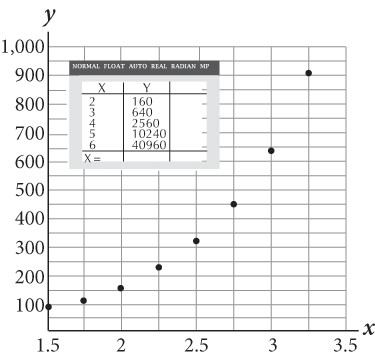# SAT Math Multiple Choice Question 531: Answer and Explanation

### Test Information

Question: 531

6.If an exponential function is used to model the data shown in the figure, and it is written in the form f(x) = f(0)(1 + r)x, what would be the value of r?

• A. 2
• B. 3
• C. 4
• D. 5